# Leibniz-Hopf algebra

(diff) ← Older revision | Latest revision (diff) | Newer revision → (diff)

Letbe the free associative algebra on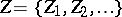over the integers. Givea Hopf algebra structure by means of the following co-multiplication, augmentation, and antipode:where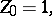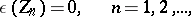where the sum is over all strings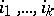,, such that. This makes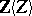a Hopf algebra, called the Leibniz–Hopf algebra. This Hopf algebra is important, e.g., in the theory of curves of non-commutative formal groups (see Formal group) [a1], [a2], [a5]. Its commutative quotient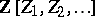, with the same co-multiplication, is the underlying Hopf algebra of the (big) Witt vector functor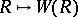(see Witt vector) and it plays an important role in the classification theory of unipotent commutative algebraic groups and in the theory of commutative formal groups (amongst other things) [a3].

The Leibniz–Hopf algebrais free as a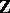-module and graded. Its graded dual is also a Hopf algebra, whose underlying algebra is the overlapping shuffle algebra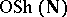. As a-module,is free with basis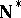, the free monoid (see Free semi-group) of all words in the alphabetwith the duality pairing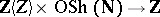given by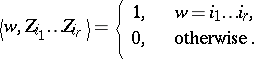The overlapping shuffle product of two such words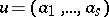,is equal to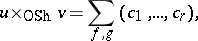where the sum is over all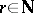and pairs of order-preserving injective mappings,such that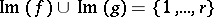, and where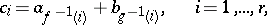with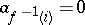if, and similarly for.

For example,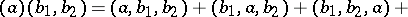The terms of maximal length of the overlapping shuffle product form the shuffle product, see Shuffle algebra.

A word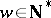,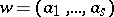, is elementary if the greatest common divisor of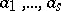is. With this terminology, the Ditters–Scholtens theorem [a4], [a5] says that, as an algebra over, the overlapping shuffle algebrais the free commutative polynomial algebra with as generators the elementary concatenation powers of elementary Lyndon words (see Lyndon word). (E.g., the third concatenation power ofis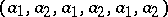.) In contrast with the case of the shuffle algebra, this theorem already holds over(not just over).

How to Cite This Entry:
Leibniz-Hopf algebra. Encyclopedia of Mathematics. URL: http://encyclopediaofmath.org/index.php?title=Leibniz-Hopf_algebra&oldid=14247
This article was adapted from an original article by M. Hazewinkel (originator), which appeared in Encyclopedia of Mathematics - ISBN 1402006098. See original article